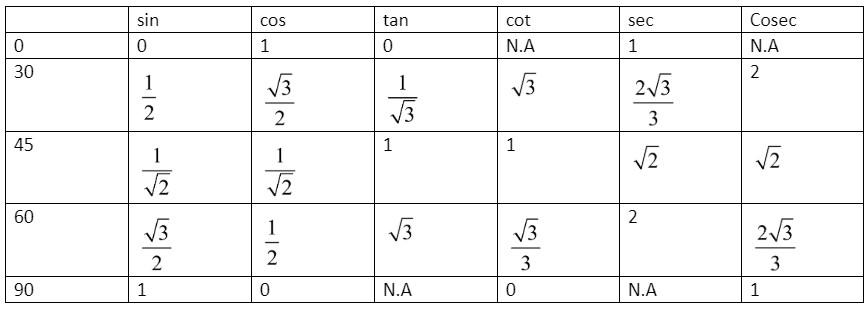Courses
Courses for Kids
Free study material
Free LIVE classes
MoreLIVE
Join Vedantu’s FREE Mastercalss

# Find the value of x, if $\tan 3x=\sin 45.\cos 45+\sin 30$.Verified
270.2k+ views
Hint: Form the table of trigonometric values with angles such as ${{0}^{\circ }},{{30}^{\circ }},{{45}^{\circ }},{{60}^{\circ }},{{90}^{\circ }}$and with trigonometric function sine, cosine, tangent. Find the value of trigonometric functions from the question and substitute to find the required answer.

Given, $\tan 3x=\sin 45.\cos 45+\sin 30$.
We can find the values of RHS using basic trigonometric formulas.
We can find it by creating a trigonometric table with the required angles such as ${{0}^{\circ }},{{30}^{\circ }},{{45}^{\circ }},{{60}^{\circ }}$and ${{90}^{\circ }}$. With 6 trigonometric functions such as sine, cosine, tangent, cosecant, secant and cotangent.
We can draw the table.Now considering the RHS = $\sin 45.\cos 45+\sin 30$.
From the above table we can find the values of sin45, cos45 and sin30.
Value of $\sin 45=\dfrac{1}{\sqrt{2}}$.
Value of $\cos 45=\dfrac{1}{\sqrt{2}}$.
Value of $\sin 30=\dfrac{1}{2}$.
Substituting the values in RHS we get,
\begin{align} & RHS=\sin 45.\cos 45+\sin 30 \\ & =\dfrac{1}{\sqrt{2}}\times \dfrac{1}{\sqrt{2}}+\dfrac{1}{2}=\dfrac{1}{2}+\dfrac{1}{2}=\dfrac{2}{2}=1 \\ \end{align}
Given, $LHS=\tan 3x$, putting the value of RHS, we get,
\begin{align} & \tan 3x=1 \\ & \Rightarrow 3x{{\tan }^{-1}}\left( 1 \right) \\ \end{align}
From the table, $\tan 45=1$.
Taking the ${{\tan }^{-1}}\left( 1 \right)$we get ${{45}^{\circ }}$.
\begin{align} & \therefore 3x=45 \\ & \Rightarrow x=\dfrac{45}{3}={{15}^{\circ }} \\ \end{align}
Therefore, we get the values of x as ${{15}^{\circ }}$.
Note:
We got ${{\tan }^{-1}}\left( 1 \right)={{45}^{\circ }}$.
We know ${{\tan }^{-1}}\theta =\cot \theta$i.e. $\dfrac{1}{\tan \theta }=\cot \theta$.
From the table of the trigonometric functions. Or try to remember the first 3 functions $\sin \theta ,\cos \theta$ and $\tan \theta$. The other 3 functions can be found taking the reverse of $\sin \theta$ (to get $\cos ec\theta$), $\cos \theta$ (to get $\sec \theta$) and $\tan \theta$ (to get $\cot \theta$).
Last updated date: 30th Sep 2023
Total views: 270.2k
Views today: 7.70k